# Can You Get a Negative out of a Square Root?

Unmatched math delimiters. Adding final one for you.

The simple answer is: yes you can get negative numbers out of square roots. In fact, should you wish to find the square root of any positive real numbers, you will get two results: the positive and negative versions of the same number.

## Writing a Square Root Equation for Positive and Negative Results

Consider the following:

16 = 4 * 4 = (-4) * (-4)

In the equation above, you can either multiply 4 by itself or multiply (-4) by itself to get the result of 16. Thus, the square root of 16 would be: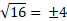The √ symbol is called the radical symbol, while the number or expression inside the symbol—in this case, 16—is called a radicand.

Why would we need a ± symbol in front of 4? Well, as we have discussed before, the square roots of 16 can be either 4 or (-4)​.

Most people would simply write this equation simply as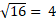. While it is technically true and there’s nothing wrong with it, it doesn’t tell the whole story.

Instead, you can also write the equation in such a way that it explicitly indicates that you want both the positive and negative square root’s results: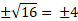This way, other people can easily tell that the one who writes the equation wishes to have positive and negative numbers as the result.

## Perfect and Imperfect squares

As you may know, 16 is a perfect square. Perfect squares are radicands in the form of an integer, or a whole number, that has a square root of another integer. In the example above, 16 is a perfect square because it has the number 4 as its square root.

Positive real numbers are not always perfect squares. There are also other numbers such as 3, 5, or 13 that are referred to as imperfect squares. If a radicand is not a perfect square, then the square root of the radicand won’t result is an integer. Take a look at the equation below.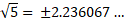5 is not a perfect square. Therefore, its square root won’t be an integer. Additionally, the square root is not even a rational number. Rational numbers are numbers that can be expressed as fractions composed of two integers, e.g. 7/2​, 50/4​, and 100/3​.

The square root of 5 is an irrational number since it can’t be expressed as fractions. The numbers right of the decimal 2.236067 … would continue on endlessly without any repeating pattern. Still, both irrational and rational numbers are part of real numbers, meaning they have tangible values and exist on the number line.

## Square Roots of Zero and Negative Numbers

We mentioned earlier that any positive real numbers have two square roots, the positive one and the negative one. What about negative numbers and zero?

For zero, it only has one square root, which is itself, 0.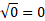On the other hand, negative numbers don’t have any real square roots. Any real number—whether it’s positive or negative—that is multiplied by itself is always equal to a positive number, except for 0. Instead, the square root of all negative numbers is an imaginary number.or

By definition, the square root of (-1)​ is i​, which is an imaginary unit. As a side note, imaginary numbers do not have a tangible value. They are not part of real numbers in the sense that they can’t be quantified on the number line. However, they are still used in math and the study of sciences including quantum mechanics, electricity, and more.

To get a better understanding, let’s take a look at an example. For instance, let’s say we want to identify the square root of (-9)​, what would it be?